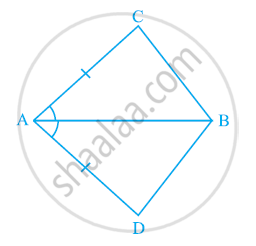# In quadrilateral ACBD, AC = AD and AB bisects ∠A (See the given figure). Show that ΔABC ≅ ΔABD. What can you say about BC and BD? - Mathematics

In quadrilateral ACBD, AC = AD and AB bisects ∠A (See the given figure). Show that ΔABC ≅ ΔABD. What can you say about BC and BD?#### Solution

In ΔABC and ΔABD,

∠CAB = ∠DAB (AB bisects ∠A)

AB = AB (Common)

∴ ΔABC ≅ ΔABD (By SAS congruence rule)

∴ BC = BD (By CPCT)

Therefore, BC and BD are of equal lengths.

Concept: Criteria for Congruence of Triangles
Is there an error in this question or solution?
Chapter 7: Triangles - Exercise 7.1 [Page 118]

#### APPEARS IN

NCERT Class 9 Maths
Chapter 7 Triangles
Exercise 7.1 | Q 1 | Page 118
Share